# Envelope approximations for global optimization

Tags:

Updated:

The global solver BMIBNB is a YALMIP-based implementation of a standard spatial branch-and-bound strategy. If you are unfamiliar with the basic ideas in a branch-and-bound solver, you should try to find an introduction first.

A spatial branch-and-bound algorithm for nonconvex programming typically relies on a few standard steps.

1. The starting open node is the original optimization problem.
2. In an open node, a local nonlinear solver is applied and a feasible, and hopefully locally optimal solution is computed. This gives an upper bound on the achievable objective (possibly infinite if the solver fails to find a feasible solution). The local solver for this step is specified with the option ‘bmibnb.uppersolver’ in BMIBNB.
3. As a second step a convex relaxation of the model in the node is derived (using the methods described below), and the resulting convex optimization problem is solved (typically a linear program, or if the original problem is a nonconvex semidefinite program, a semidefinite program). This gives a lower bound on the achievable objective for this node. The lower bound solver is specified using the options ‘bmibnb.lowersolver’ in BMIBNB.
4. Given these lower and upper bounds, a standard branch-and-bound logic is used to select a branch variable, create two new nodes, branch, prune and navigate among the remaining nodes.

In addition to these standard steps, a large amount of preprocessing and bound-propagation is performed, both in the root-node and along the branching. This is important in order to obtain stronger linear relaxations as we will see below. The options controlling this can be found in the description of BMIBNB. Nevertheless, the central object is the relaxation, and this model is built using outer approximations of convex envelopes (the convex hull of the set $$(x,f(x))$$ on some interval in $$x$$).

### Linear relaxation for bilinear and quadratic problems

The solver implements a standard relaxation strategy where all bilinear and quadratic terms are replaced with new linear variables. Based on bounds on the variables in the term, constraints are added to relate the new linear variable with the original nonlinear term, in order to make the relaxation as tight as possible.

Given two variables $$x$$ and $$y$$, and lower and upper bounds $$x_L$$, $$x_U$$, $$y_L$$, $$y_U$$, the following inequalities trivially hold McCormick 1976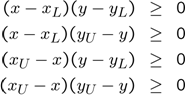A linear relaxation of the nonlinear monomials are obtained by introducing new variables $$w_x$$, $$w_{xy}$$ and $$w_y$$, and replacing $$x^2$$, $$xy$$ and $$y^2$$ in the constraints above and the original model with these $$w$$ variables. A set of linear equalities is then obtained. This procedure is applied to all nonlinear terms.

We can use YALMIP to illustrate this for a simple scalar nonlinearity $$x^2$$. We generate the nonlinear representation of the generating equations

sdpvar x;
xL = -1;
xU = 2;
constraints =[(xU-x)*(x-xL)>=0,(xU-x)*(xU-x)>=0,(x-xL)*(x-xL)>=0]


A linear relaxation is obtained by introducing a new variable, and using this variable instead of $$x^2$$. This is essentially what happens when you use the option relax when you solve optimization problems. Any monomial term is treated as an independent variable. We use this trick to easily plot the linear relaxation.

plot(constraints,[x;x^2],[],[],sdpsettings('relax',1))
t = (-1:0.01:2);
hold on;plot(t,t.^2);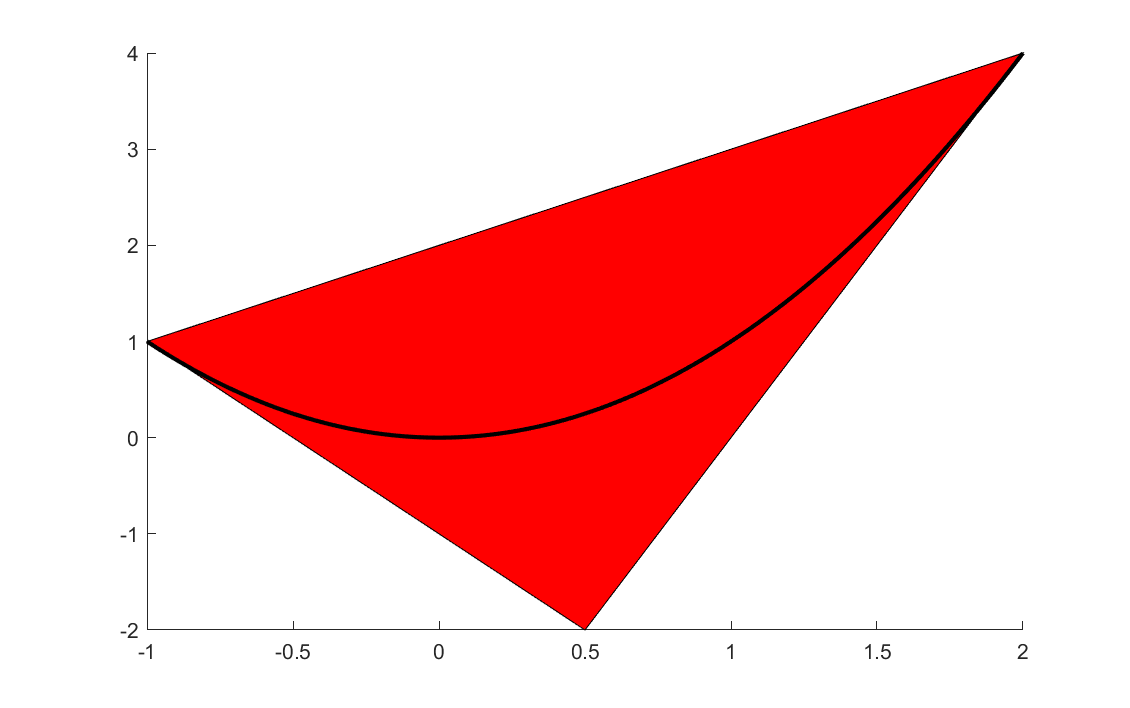The horizontal axis is the original variable $$x$$ and the vertical is the relaxed variable representing $$x^2$$, and the polytope shows the feasible region for the relaxed variable. Clearly, the approximation is perfect at the bounds, but fairly poor in the middle. It should be stated that non-negativity on variables representing quadratic terms automatically are appended in the envelope implementation, thus cutting off the lower negative portion of the polytope (see below)

By tightening the bounds during the branching process, the linear relaxation will become increasingly better. As an example, if the solver branches on $$x$$ at 0.5, two new nodes would be created with stronger relaxations.

sdpvar x;
xL1 = -1;
xU1 = 0.5;
xL2 = 0.5;
xU2 = 2;

constraints1 =[(xU1-x)*(x-xL1)>=0,(xU1-x)*(xU1-x)>=0,(x-xL1)*(x-xL1)>=0]
constraints2 =[(xU2-x)*(x-xL2)>=0,(xU2-x)*(xU2-x)>=0,(x-xL2)*(x-xL2)>=0]

clf
plot(constraints1,[x;x^2],[],[],sdpsettings('relax',1))
hold on
plot(constraints2,[x;x^2],[],[],sdpsettings('relax',1))
t = (-1:0.01:2);
hold on;plot(t,t.^2);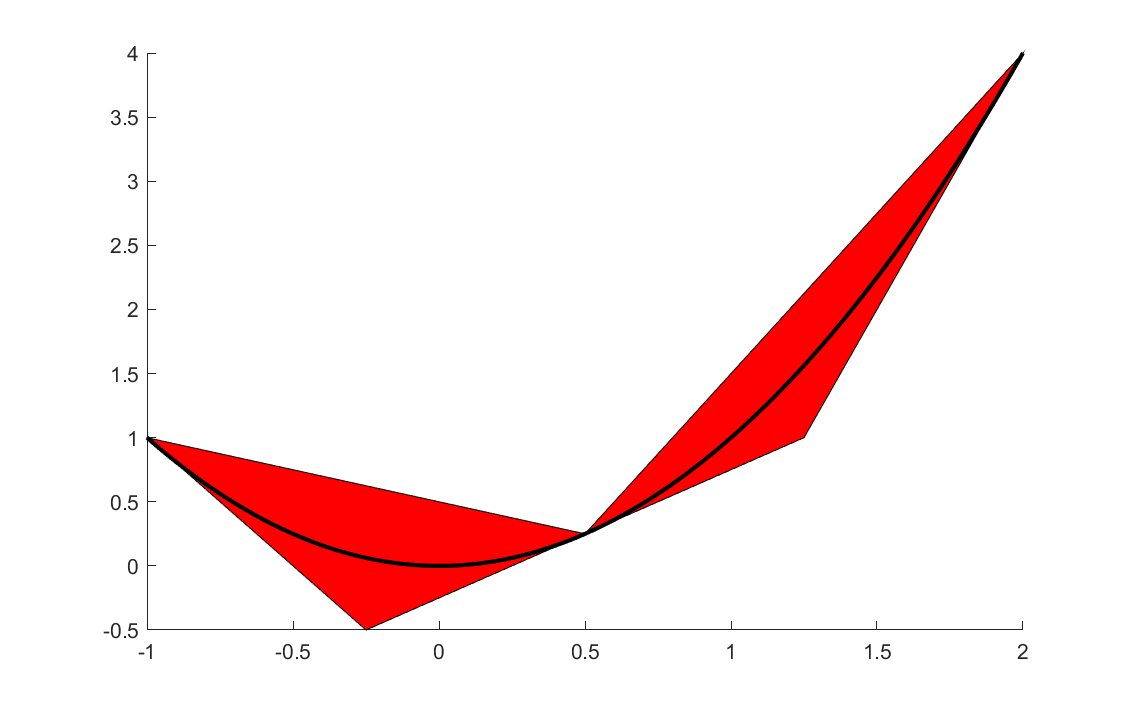Note that the algorithm requires bounds on the variables. If YALMIP fails to extract any explicit bounds from the model in the root-node, the code will almost always terminate and an error message will be displayed. Addionally, the tighter the bounds are, i.e., closer to the final optimal value, the better the envelope approximation will be.

### Linear relaxation for polynomial problems

Multivariate polynomial problems are treated by simply converting them to bilinear problems, by introducing additional variables and constraints. As an example, the term $$x^2y^2$$ can be written as $$uv$$ with the new bilinear constraints $$u=x^2$$ and $$v=y^2$$. Once a bilinear model has been obtained, standard bilinear relaxations can be used.

### Linear relaxation for general problems

As in the polynomial case, every nonlinear scalar term $$f(x)$$ is replaced with a new variable $$w$$, and linear inequalities on $$x$$ and $$w$$ are introduced to ensure that $$w$$ approximates $$f(x)$$ well, i.e., that the curve $$w = f(x)$$ is contained in the polytopic region in $$A_x x + A_w w \leq b$$.

As an example, for the sqrtm function, the following cut is used internally (for a concave function, the convex hull approximation is automatically generated by a lower hyperplane joining the function values at the interval end-points, and two upper hyperplanes defined by the tangents at the end-points)

xL = 0.1;
xU = 3;
Aw = [1; 1; -1];
xM = (xL + xU)/2;
b = [sqrt(xL) - xL/(2*sqrt(xL));
sqrt(xU) - xU/(2*sqrt(xU));
sqrt(xU)*(xL)/(xU-xL) -  sqrt(xL)*(xU)/(xU-xL)];

Ax = [-1/sqrt(4*xL);
-1/sqrt(4*xU);
sqrt(xU)/(xU-xL) - sqrt(xL)/(xU-xL)];
sdpvar x w
figure
plot([Ax*x + Aw*w <= b],[x;w]);
t = (xL:0.01:xU);
hold on;plot(t,sqrt(t));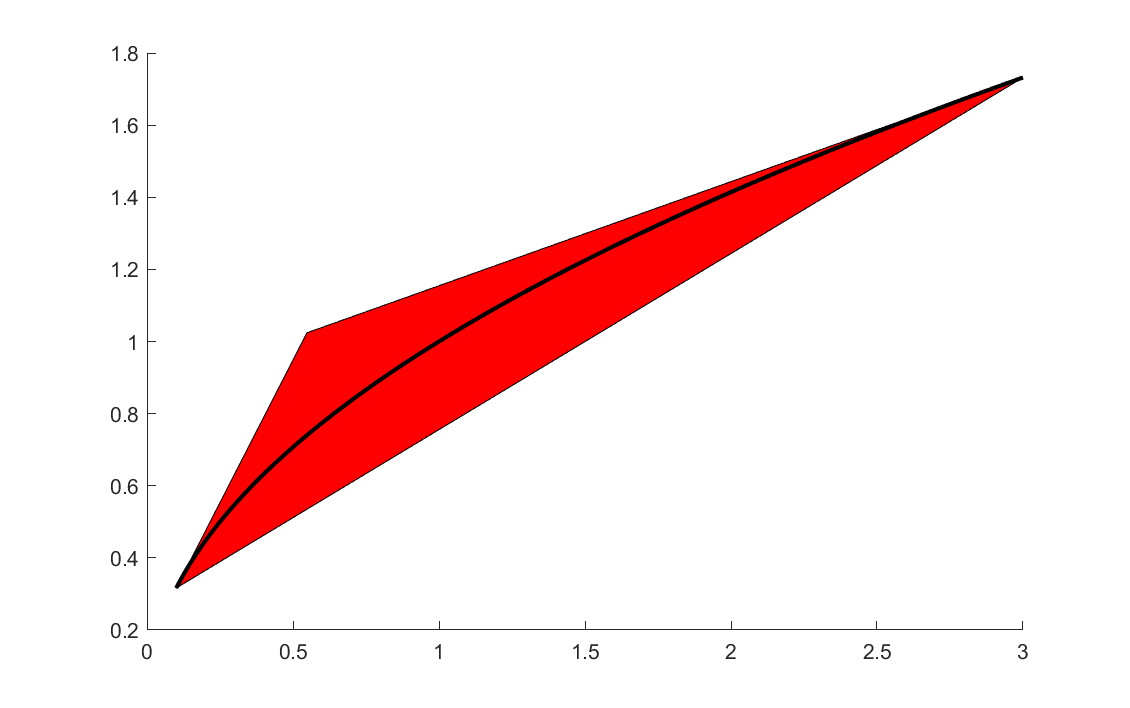Explicit representations of the envelopes are implemented for most nonlinear operators, such as x^p, exp, log and sqrtm. For convex and concave functions no dedicated envelope code is required as long derivatives are available, as an envelope approximation can be dervied from gradients. For some other functions, and sdpfun based operators, a sampling strategy is used to derive the linear relaxation. As an example, the following code computes an approximation of the convex envelope of sin with three facets.

xL = 0;
xU = 3*pi/2;
z = linspace(xL,xU,100);
fz = sin(z);
k1 = max((fz(2:end)-fz(1))./(z(2:end)-xL))+1e-3;
k2 = min((fz(2:end)-fz(1))./(z(2:end)-xL))-1e-3;
k3 = min((fz(1:end-1)-fz(end))./(z(1:end-1)-xU))+1e-3;
k4 = max((fz(1:end-1)-fz(end))./(z(1:end-1)-xU))-1e-3;
Ax = [-k1;k2;-k3;k4];
Aw = [1;-1;1;-1];
b =  [k1*(-z(1)) + fz(1);-(k2*(-z(1)) + fz(1));k3*(-z(end)) + fz(end);-(k4*(-z(end)) + fz(end))];
sdpvar x w
figure
plot([Ax*x + Aw*w <= b],[x;w]);
t = (xL:0.01:xU);
hold on;plot(t,sin(t));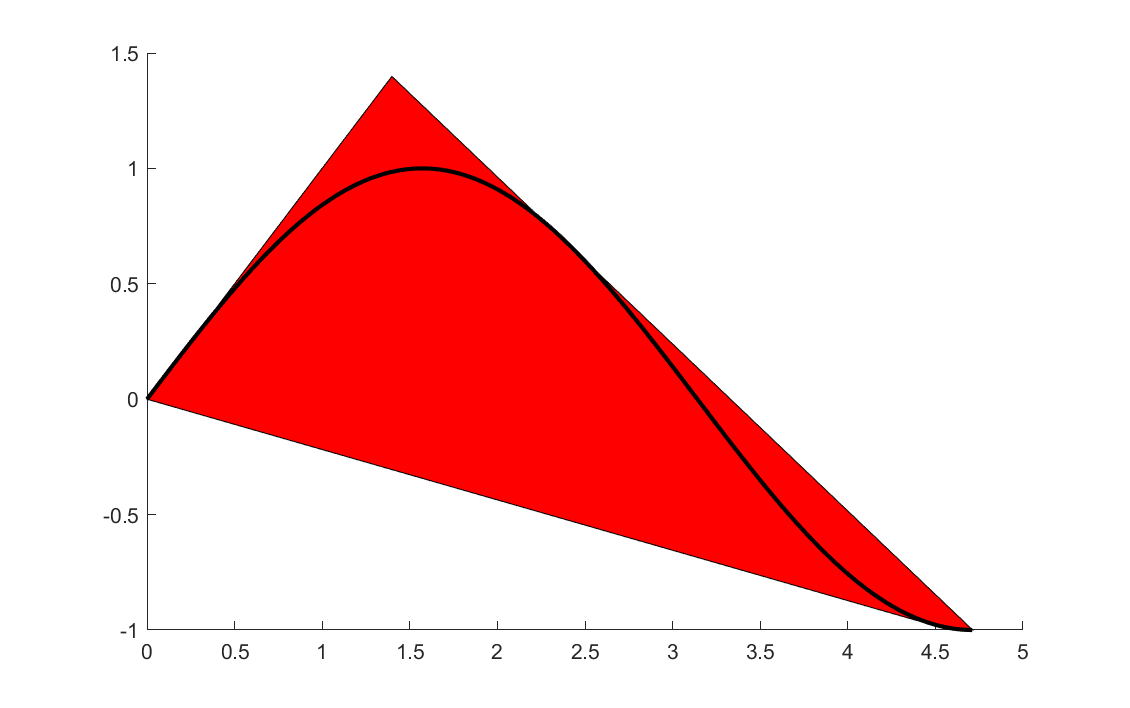### Exploiting YALMIPs internal framework

In the code above, we generated the envelope approximations manually, but it is of course possible to hook into the inner workings of YALMIP to study these sets.

Our sin example

sdpvar w x
E = envelope([0 <= x <= 3*pi/2, w == sin(x)]);
plot(E,[x;w],[],[],sdpsettings('relax',1));
hold on
x = linspace(0,3*pi/2,100);
plot(x,sin(x))


Note that the envelope object E still contains the sin(x) variable (in practice it can be eliminated and replaced with w, but for implementation purposes it is kept in the form above with a trivial equality in the model), hence we must tell YALMIP to relax all variables, and then plot the projection to the $$x,w$$ plane.

If you study the quadratic monomial from earlier, you will see that YALMIP not only adds a positivity cut to the quadratic envelope, but also adds three tangency cuts. How many cuts BMIBNB uses is basically a trade-off between tightness of the relaxations, and the computational effort needed in the lower bound solvers with additional cuts.

sdpvar w x
E = envelope([0 <= x <= 3*pi/2, w == x^2]);
plot(E,[x;w],[],[],sdpsettings('relax',1));
hold on
x = linspace(0,3*pi/2,100);
plot(x,x.^2)


Finally, a comment on bounds. As a general rule of thumb, you have to bound all variables used in nonlinear epxressions when you use the global solver BMIBNB which is based on the envelope approximations. However, YALMIP performs various bound strengthening schemes to improve the bounds, and the same code is used in the envelope code used above. In some cases, YALMIP can derive bounds, without any initial bounds being specified at all. As an example, the following problem is easily solved with nicely behaved envelopes despite supplying no bounds

sdpvar x
optimize([x + x^2 + x^4 == 0],x,sdpsettings('solver','bmibnb'));


YALMIP easily derives the initial bound $$-1 \leq x \leq 0$$ and then improves it further to $$-0.6875 \leq x \leq 0$$ (the optimal solution is $$x=-0.6823$$, so the lower bound is essentially tight), and can use this when creating the cuts for the envelope approximation. A bit silly plot, but you will get a line in $$x$$ here showing the feasible interval.

E = envelope([x + x^2 + x^4 == w, w == 0]);
plot(E,[x;w],[],[],sdpsettings('relax',1));


Can you also derive the interval from the nonlinear equation?